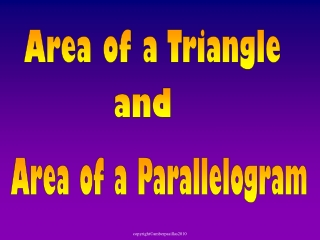DownloadDownload PresentationArea of a Parallelogram

# Area of a Parallelogram

Download Presentation## Area of a Parallelogram

- - - - - - - - - - - - - - - - - - - - - - - - - - - E N D - - - - - - - - - - - - - - - - - - - - - - - - - - -
##### Presentation Transcript

1. Area of a Triangle and Area of a Parallelogram

2. Polygons Today we are going to find the Area ofParallelogramsand the Area ofTriangles

3. Polygons Area The number of square units that are needed to cover the surface of a figure. Polygon Any straight-sided closed plane figure.

4. Area of a Rectangle The area of a rectangle is equal to the base times the height. Also known as length times width. height A = bh (h) (b) base A = bh is the same as A = lw

5. Area of a Rectangle What is the area of the rectangle? 2 2 in. x 6 12 in.2 6 in. 12 in2 or square inches

6. Area of a Square A square is a special rectangle. Since the base and the height are the same size, we call them sides (s) instead of base and height. A= s2 s height = s s base = s

7. Area of a Square What is the area of the square? 4 4 m. 4 x 16 m.2 4 m. 16m2 or square meters

8. Area of a Parallelogram Given the formula for area of a rectangle, we are going to use that information to derive the formula for the area of a parallelogram Watch carefully not to miss it!

9. Area of a Parallelogram Draw a straight line from the top corner perpendicular to the base Cut that triangle and move it to the other side What shape does it make? Rectangle

10. Area of a Parallelogram Use this information to find thearea of a parallelogram h height = h h b base = b A parallelogram has the same area as a rectangle! What is the formula forarea of a parallelogram?

11. Area of a Parallelogram 2 cm. 90º 4 cm. = 8 cm.2 Check to see if you got it right.

12. Area of a Parallelogram 2 cm. 90º 4 cm. = 8 cm.2 Cut off the piece at the dotted line.

13. Area of a Parallelogram 2 cm. 90º 4 cm. = 8 cm.2 Cut off the piece at the dotted line.

14. Area of a Parallelogram 2 cm. 90º 4 cm. = 8 cm.2 Move this piece to the other side.

15. Area of a Parallelogram 2 cm. 90º 4 cm. = 8 cm.2 Move this piece to the other side.

16. Area of a Parallelogram Let’s check it with the area of a rectangle. 2 cm. 90º 4 cm. = 8 cm.2 Now you have a rectangle How many squares do you see? A = 8square cm. or 8 cm.2

17. Area of a Parallelogram What is the area of this parallelogram? 5 5 cm. 7 x 35 cm.2 7 cm. 35 square centimeters or 35 cm.2

18. Area of a Parallelogram What is the area of this parallelogram? 5 6 x 5 ft. 30 ft.2 6 ft. 30 square feet or 30 ft.2

19. Area of a Triangle Given the formula for area of a rectangle, we are going to use that information to discover the formula for the area of a triangle. Watch carefully not to miss it!

20. Area of a Triangle Given a right triangle Make a similar triangle,

21. Area of a Triangle Given a right triangle What polygon is this? A Rectangle Make a similar triangle, and put both triangles next to each other flip it

22. Area of a Triangle We can use the formula for area of a rectangle to find the formula for area of a triangle. Twotriangles make one rectangle. We want to find half of the area of the rectangle. height h base b Whatis the formula for the area of a triangle?

23. Area of a Triangle When we put 2 right triangles together is made a rectangle. Watch what happens when instead we use 2 isosceles triangles.

24. Area of a Triangle Given an isosceles triangle Make a similar triangle,

25. Area of a Triangle Given an isosceles triangle What polygon is this? A Parallelogram Make a similar triangle, and put both triangles next to each other flip it

26. height h base Area of a Triangle How do you find the area of the parallelogram?

27. Area of a Triangle 5 cm 6 cm 3 cm 9 cm

28. Area of a Triangle The End! Take out your study guide!

29. Area of a Parallelogram # 3 To find the area for a parallelogram use what you know about area of a rectangle. A = base x height 3 in A = b x h 5 in A = 5 x 3 = 15 in2

30. Area of a Triangle # 4 A triangle is half the area of a rectangle. To find the area of a triangle you use the rectangle formula and divide it in half. A = base x height 2 6 m 8 m A = 8 x 6 = 24 m2 2